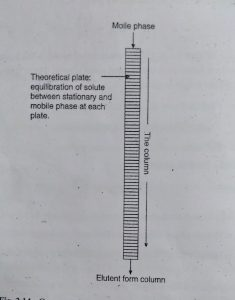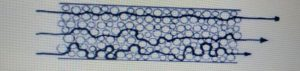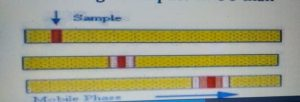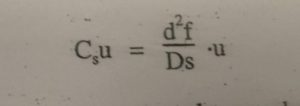# Chromatography:Plate and Rate Theory and MCQ based on GPAT, GATE, UGC NET JRF Competitive Exams Sciences Stream

## Chromatography:Plate and Rate Theory and MCQ based on GPAT, GATE, UGC NET JRF Competitive Exams Sciences Stream

There are mainly two theory for column Chromatography is

• 1. Plate Theory
• 2. Rate Theory

Plate Theory :-

Plate Theory is given by martin and synge in 1941.

Plate Theory is useful for understanding the mechanism of retention and transfer of analyte through the column length.

This Theory is based on the assumption that column is consists of the large number of plate called as Theoretical Plate.

At each plate separate equilibration of analyte between stationary and mobile phase is achieved.Column efficiency can be explained by two terms plate height (H) and plate count or plate number (N).

N=L/H it is also written as

HETP = L/N

Where,

HETP = Height Equivalent to a theoretical plate

L = length

N = Number of theoretical plate.

Martin and synge given two term

1. Retention time

2. Peak width (W)

N= 16(tR/W)²

Where,

tR = retention time

W = peak width

N= number of plate.

Retention time is the time required to elute out peak maximum for solute from the point of injection or time required to elute out half of the solute from the point of injection.

HETP is minimum height to required equal distribution of analyte and mobile phase on the stationary phase.

2. Rate Theory :-

It was introduced by van Deemter.

It describes the effect of an Elution band as well as it time of Elution.

Van Deemter equation describe the relationship of the height of a theoretical plate H and the average linear velocity of the Mobile phase.

Vandeemter equation :-

H = A+ B/μ+ cμ

Where,

H = Height of a theoretical plate

μ = average of velocity of mobile phase

A = eddy diffusion

B= longtiudinal diffusion

C=  Non – equilibrium or resistance to mass transfer term.

• Eddy Diffusion :-

The term refers to band broadening caused by multi pathway effect

A= 2λdp

Where ,

λ = correction factor for the irregular of the column packing

dP = average particle diameter

In this case the space along the column are not uniform.

When a sample is migrate down the column each molecule “sees” different path and each path is of a different length.

Some molecules take the longer paths and other take the shorter path.• Longitudinal Diffusion

The term B represent band broadening by longitudinal diffusion the molecular diffusion both in and against the flow direction :

B= 2yDG

Where,

y = labyrinth factor of the pore channel

DG = diffusion coefficient of the Analyte in the gas phase

This process result when there exists a region of high concentration and a region of low concentration.

The migration is from the higher to the lower concentration region in the axial direction of the column

Diffusion occur on the molecular level resulting from movement of molecules after collision.

The diffusion is about 100- 1000 fold faster in gas than in liquid therefore B term shows higher impact in GC than in LC.Resistance to mass transfer :-In case of thick films, solutes must travel to reach the interface. With smaller diffusion coefficient these solute molecules travel more slowly. These factor lead to lower rate of mass transfer and plate height is increased.

df= film thickness of liquid s.p.

μ = Average linear velocity of mobile phase.

Ds = Diffusion co efficient in s.p.

Reference: text book of P.analysis of third edition Dr.s.ravi sankar

×
Free Video Lectures of Pharmacy Exams
Apply now